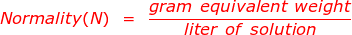# Normality Formula

Normality Formula

Normality is a rarely used expression which indicates the concentration of a solution. It is defined as the gram equivalent weight per liter of solution.The reason normality is rarely used lies in the definition of gram equivalent weight. Gram equivalent weight is determined by the amount of an ion that reacts, which could change depending on the reaction. The fact that the gram equivalent weight is not a consistent amount creates confusion and leads scientists to use other concentration values.

Normality, when used, is typically done so in acid base reactions. Here, a more favorable calculation for normality is considered, where the normality of a solution is equal to the molarity multiplied by the number of equivalents.

Normality(N) = Molarity(M) x number of equivalents

Normality Formula Questions:

1. What is the normality of 1.0 M H2SO4 in the following reaction?

H2SO4 + 2NaOH → Na2SO4 + 2H2O

In this reaction, 2 moles of H+ ions (2 equivalents)from sulfuric acid (H2SO4) react with NaOH to form sodium sulfate and water.

Normality(N) = Molarity(M) x number of equivalents

Normality(N) = 1.0 x 2

Normality(N) = 2.0

2. What is the normality of 1.0 M H3PO4 in the following reaction?

H3AsO4 + 2NaOH → Na2HAsO4 + 2H2O

In this reaction, only 2 of the three available H+ ions (2 equivalents) from arsenic acid (H3AsO4) react with NaOH to form Na2HAsO4 and water.

Normality(N) = Molarity(M) x number of equivalents

Normality(N) = 1.0 x 2

Normality(N) = 2.0